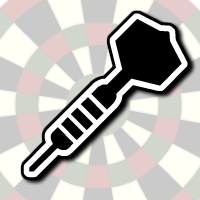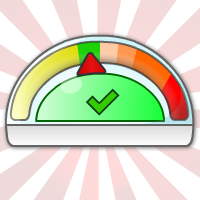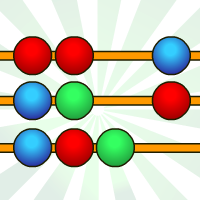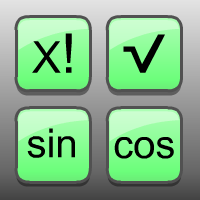# 35,000 BCE - Early Humans

The first signs of mathematics can be traced back to the prehistoric era, where early humans used basic counting methods to keep track of quantities such as food, animals, and possessions. Archaeologists have discovered evidence of tally sticks and bone artifacts that suggest that early humans used simple counting systems as far back as 35,000 BCE.

# 3000 BCE - The Egyptians

The ancient Egyptians are credited with developing one of the earliest mathematical systems, which dates back to around 3000 BCE. The Egyptians used a decimal system of numeration based on the number 10, which they used to solve practical problems such as land surveying and taxation.

The Egyptians also developed a system of hieroglyphic symbols to represent numbers, which they used to record mathematical calculations on papyrus scrolls. They were able to perform arithmetic operations such as addition, subtraction, multiplication, and division, as well as solve basic algebraic equations.

The Egyptians' mathematical knowledge was passed down through the ages and influenced the development of mathematics in other ancient civilizations, such as the Greeks and Babylonians. The legacy of Egyptian mathematics can still be seen in modern-day mathematical systems, particularly in the use of fractions and the concept of place value.

# 400 BCE - The Greeks

The ancient Greeks developed mathematics over a period of several centuries, from around the 6th century BCE to the 4th century BCE. Greek mathematics is known for its emphasis on geometry, which was developed by the mathematician Euclid in his influential work "Elements". The "Elements" is a comprehensive treatise on geometry that covers a wide range of topics, including basic principles, plane geometry, solid geometry, and number theory.

The Greeks also made important contributions to other branches of mathematics, such as algebra and number theory. The mathematician Pythagoras, for example, is credited with discovering the Pythagorean theorem, which is a fundamental concept in geometry. Another important Greek mathematician was Archimedes, who made significant advances in calculus, geometry, and mechanics.

# 14th Century - Islamic Golden Age

During the Islamic Golden Age, which lasted from the 8th to the 14th century, Islamic scholars made significant contributions to mathematics. They translated Greek and Indian mathematical texts into Arabic and made important advances in algebra, trigonometry, and geometry. The Persian mathematician Al-Khwarizmi, for example, wrote a treatise on algebra that introduced the concept of algebraic equations.

In the 16th and 17th centuries, mathematicians such as Johannes Kepler and Galileo Galilei used mathematics to explain physical phenomena and develop new scientific theories. This led to the development of calculus, which was independently invented by Isaac Newton and Gottfried Leibniz. Calculus is a branch of mathematics that deals with the study of rates of change and the accumulation of small increments.

# 16th Century - The First Calculator

The first calculator-like device was invented in the 17th century and was known as the Pascaline, after its inventor, the French mathematician Blaise Pascal. The Pascaline was designed to help his father, a tax collector, perform complex calculations more quickly and accurately.

The Pascaline was a simple mechanical device that used a series of gears and wheels to add and subtract numbers. It could perform calculations up to eight digits long and was capable of carrying numbers over from one digit to the next. The Pascaline was operated by turning a crank, which rotated the wheels and gears inside the device.

Although the Pascaline was a significant step forward in the development of calculating devices, it was expensive to produce and not widely adopted. Other inventors, such as the German mathematician and philosopher Gottfried Wilhelm Leibniz, also developed similar machines around the same time.

# 19th Century - Calculators

The 19th century saw significant advancements in the development of mechanical calculators. In 1820, the French mathematician Charles Xavier Thomas de Colmar invented the Arithmometer, which was the first commercially successful mechanical calculator. The Arithmometer used a series of rotating cylinders with digits on them to perform addition, subtraction, multiplication, and division.

Other inventors, such as the German mathematician and inventor Georg Scheutz, also made significant contributions to the development of mechanical calculators. Scheutz invented the first successful mechanical calculator that could perform complex mathematical operations such as logarithms and trigonometric functions.

During the second half of the 19th century, several companies began producing mechanical calculators on a large scale. One of the most famous of these was the Comptometer, which was invented by the American businessman Dorr Eugene Felt in 1887. The Comptometer was widely used in businesses and government offices for many decades and was one of the first calculators to use a keyboard instead of rotating cylinders or wheels.

# 20th Century - Digital Calculators

The 20th century saw significant advancements in the development of electronic and digital calculators, which revolutionized the way we perform calculations. The first electronic calculator, known as the Bell Punch ANITA Mark VII, was developed in 1961 by the British company Bell Punch Co. It used vacuum tubes to perform basic arithmetic calculations and was relatively large and expensive.

In the 1960s and 1970s, several companies, including Texas Instruments and Hewlett-Packard, developed smaller and more affordable electronic calculators. These calculators used solid-state transistors and integrated circuits to perform calculations and were much faster and more reliable than their mechanical counterparts.

In the 1970s and 1980s, pocket calculators became increasingly popular and affordable, making them accessible to a wider range of people. These calculators were small enough to fit in a pocket or purse and could perform a wide range of calculations, including scientific and statistical functions.

In the 1990s and 2000s, digital calculators became even more advanced, with the development of graphing calculators that could display graphs and charts and programmable calculators that could perform complex mathematical functions and store user programs.

It was also the time when Online Calcualtors became a thing!

# The Future of Calculators

The future of calculators is likely to continue to evolve with advancements in technology. One trend that is likely to continue is the integration of calculators into other devices, such as smartphones, tablets, and computers. Many of these devices already have built-in calculator functions, and as technology continues to improve, we may see even more sophisticated and powerful calculators integrated into these devices.

Another trend is the development of more specialized calculators designed for specific fields and applications. For example, there are already calculators designed specifically for use in finance, engineering, and science, and we may see more of these types of calculators in the future.

The use of artificial intelligence and machine learning may also play a role in the development of calculators. For example, we may see calculators that can analyze data and make predictions based on historical trends, or calculators that can suggest the best approach to solving a particular problem based on the user's input.

Overall, the future of calculators is likely to be shaped by technological advancements and the needs of specific industries and applications. As these needs continue to evolve, we can expect calculators to become even more sophisticated and capable of performing increasingly complex calculations and functions.

# Conversions Calculators

## Effortlessly convert units, currencies, and more with our comprehensive range of simple to use conversion calculators! Here are just a few, make sure you check our all the others we have to offer!

Our weight conversion tools are accurate, easy to use, and offer a wide range of units.
Get precise and hassle-free length conversions with our user-friendly and comprehensive length conversion calculators.
Our temperature conversion calculators are accurate, simple to use, and covers various temperature units.
Loads of other easy to use conversion tools!

## Featured Tools:

These are some of the most popular calculators and tools on the site!### Darts Calculator

Our Darts Calculator helps players calculate scores and checkouts quickly and accurately, making the game more enjoyable and competitive!

Darts Calculator### BMI Calculator

Our BMI Calculator quickly calculates Body Mass Index to help users understand their weight status and make informed health decisions.

BMI Calculator### Online Abacus

Our Online Abacus is a virtual version of the traditional counting tool, designed to help children learn and practice basic math skills.

Online Abacus### Scientific Calculator

Our Scientific Calculator is a powerful tool that performs complex mathematical calculations and functions.

Scientific Calculator